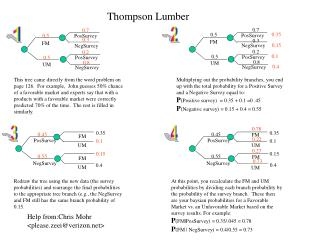DownloadDownload PresentationThompson Lumber

# Thompson Lumber

Télécharger la présentation## Thompson Lumber

- - - - - - - - - - - - - - - - - - - - - - - - - - - E N D - - - - - - - - - - - - - - - - - - - - - - - - - - -
##### Presentation Transcript

1. Thompson Lumber 0.7 0.7 1 2 0.35 0.5 PosSurvey 0.5 PosSurvey 0.3 0.3 FM FM 0.15 NegSurvey NegSurvey 0.2 0.2 0.1 0.5 PosSurvey PosSurvey 0.5 0.8 0.8 UM UM 0.4 NegSurvey NegSurvey This tree came directly from the word problem on page 126. For example, John guesses 50% chance of a favorable market and experts say that with a products with a favorable market were correctly predicted 70% of the time. The rest is filled in similarly. Multiplying out the probability branches, you end up with the total probability for a Positive Survey and a Negative Survey equal to: P(Positive survey) = 0.35 + 0.1 =0 .45 P(Negative survey) = 0.15 + 0.4 = 0.55 0.78 3 4 0.35 0.35 0.45 0.45 FM FM 0.22 PosSurvey PosSurvey 0.1 0.1 UM UM 0.27 0.15 0.15 0.55 0.55 FM FM 0.73 NegSurvey NegSurvey 0.4 0.4 UM UM Redraw the tree using the new data (the survey probabilities) and rearrange the final probabilities to the appropriate tree branch (e.g., the NegSurvey and FM still has the same branch probability of 0.15. At this point, you recalculate the FM and UM probabilities by dividing each branch probability by the probability of the survey branch. These then are your baysian probabilities for a Favorable Market vs. an Unfavorable Market based on the survey results. For example: P(FM|PosSurvey) = 0.35/.045 = 0.78P(FM | NegSurvey) = 0.4/0.55 = 0.73 Help from:Chris Mohr <please.zeei@verizon.net>